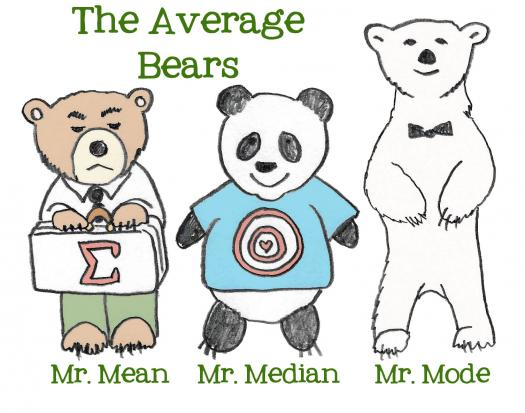# Mean, Median, And Mode

8 Questions | Total Attempts: 902SettingsMean, median, and mode are all importance mathematical concepts to understand when determining statistics. Are you a mean, median, and mode math wizard?

• 1.
What is the definition of "mean"?
• A.

The number that is repeated the most

• B.

The middle value in a number line

• C.

The average of all numbers

• 2.
How do you determine the "mean" number?
• A.

Add all numbers together and divide by the amount of numbers

• B.

Add all numbers together and multiply by four

• C.

Add all numbers together and divide by four

• D.

• 3.
What is the mean of the following numbers? 2, 22, 12, 22
• A.

22

• B.

14.5

• C.

14

• D.

12

• 4.
What is the definition of the "median"?
• A.

The middle number

• B.

The last number

• C.

The first number

• D.

The average of all numbers

• 5.
What is the median of these numbers? 3, 15, 43, 13, 52
• A.

15

• B.

13

• C.

43

• D.

3

• 6.
How do you determine the median if there is an even amount of numbers?
• A.

Choose whichever one is smaller

• B.

You cannot determine the median if there is an even amount of numbers

• C.

Add the two middle numbers and divide by two

• D.

Multiple the two middle numbers and divide by two

• 7.
What is the "mode"?
• A.

The average of all of the numbers

• B.

The middle number

• C.

The sum of all numbers together

• D.

The number that appears most frequently

• 8.
What is the most in the following number line?2, 100, 84, 43, 2, 3, 17, 84, 43, 2
• A.

84

• B.

2

• C.

43

• D.

There is not a mode

Related TopicsBack to top